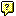## Welcome to OnlineConversion.com

Pressure Conversion

 Convert what quantity?From: atmosphere [standard] atmosphere [technical] attobar attopascal bar barad barye centibar centihg centimeter of mercury [0 °C] centimeter of water [4 °C] centipascal centitorr decibar decipascal decitorr dekabar dekapascal dyne/square centimeter exabar exapascal femtobar femtopascal foot of air [0 °C] foot of air [15 °C] foot of head foot of mercury [0 °C] foot of water [4 °C] gigabar gigapascal gram-force/square centimeter hectobar hectopascal inch of air [0 °C] inch of air [15 °C] inch of mercury [0 °C] inch of water [4 °C] kilobar kilogram-force/square centimeter kilogram-force/square meter kilogram-force/square millimeter kilonewton/square meter kilopascal kilopond/square centimeter kilopond/square meter kilopond/square millimeter kip/square foot kip/square inch megabar meganewton/square meter megapascal meter of air [0 °C] meter of air [15 °C] meter of head microbar micrometer of mercury [0 °C] micrometer of water [4 °C] micron of mercury [0 °C] micropascal millibar millihg millimeter of mercury [0 °C] millimeter of water [4 °C] millipascal millitorr nanobar nanopascal newton/square meter newton/square millimeter ounce/square inch pascal petabar petapascal picobar picopascal pieze pound/square foot pound/square inch poundal/square foot sthene/square meter technical atmosphere terabar terapascal ton/square foot [long] ton/square foot [short] ton/square inch [long] ton/square inch [short] ton/square meter torr Water Column [centimeter] Water Column [inch] Water Column [millimeter] yoctobar yoctopascal yottabar yottapascal zeptobar zeptopascal zettabar zettapascal To: atmosphere [standard] atmosphere [technical] attobar attopascal bar barad barye centibar centihg centimeter of mercury [0 °C] centimeter of water [4 °C] centipascal centitorr decibar decipascal decitorr dekabar dekapascal dyne/square centimeter exabar exapascal femtobar femtopascal foot of air [0 °C] foot of air [15 °C] foot of head foot of mercury [0 °C] foot of water [4 °C] gigabar gigapascal gram-force/square centimeter hectobar hectopascal inch of air [0 °C] inch of air [15 °C] inch of mercury [0 °C] inch of water [4 °C] kilobar kilogram-force/square centimeter kilogram-force/square meter kilogram-force/square millimeter kilonewton/square meter kilopascal kilopond/square centimeter kilopond/square meter kilopond/square millimeter kip/square foot kip/square inch megabar meganewton/square meter megapascal meter of air [0 °C] meter of air [15 °C] meter of head microbar micrometer of mercury [0 °C] micrometer of water [4 °C] micron of mercury [0 °C] micropascal millibar millihg millimeter of mercury [0 °C] millimeter of water [4 °C] millipascal millitorr nanobar nanopascal newton/square meter newton/square millimeter ounce/square inch pascal petabar petapascal picobar picopascal pieze pound/square foot pound/square inch poundal/square foot sthene/square meter technical atmosphere terabar terapascal ton/square foot [long] ton/square foot [short] ton/square inch [long] ton/square inch [short] ton/square meter torr Water Column [centimeter] Water Column [inch] Water Column [millimeter] yoctobar yoctopascal yottabar yottapascal zeptobar zeptopascal zettabar zettapascalResult:Information:
"-force" is added to distinguish units of force from units of mass.

The common PSI stands for "pounds per square inch" or "pounds/square inch".

 Did you find us useful? Please consider supporting the site with a small donation. click here for more information### BookMark Us

It may come in handy.

Check out our Conversion Software for Windows.Can't find something?
Try searching.

Are you bored?
Try the Fun Stuff.